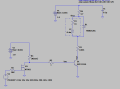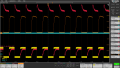# Magnetic Buzzer/transducer equivalent circuit and driver circuit optimization

#### malli_1729

Joined Nov 28, 2012
32
Hi,

I am using Magnetic buzzer into the design. Buzzer part number is SMT8585-03H03LF from Bestar electronics.

Below are the specifications for quick glance.Question1:
Is it possible to get a LTspice model for this kind of buzzer. I see an Magnetic buzzer's equivalent circuit will be Inductor in series with resister. I can get resister value from specifications but no data on coil data.

Question2:
As per testing results I have drawn an equivalent circuit as below. R2 is just extra, i am not using it in actual circuit.Here, I have 2 constraints Based max driving current which I am limiting to ~80mA,

1. With Max Ic = 80mA--> total Collector resistance ~ 40Ohms, leaving coil resistance adding 25 Ohms Collector resister - Because of the drop across this resister (25*0.080 =2V) voltage across buzzer is less ~1.2V, which is less than Min recommended voltage.
2. If i reduce the collector resistance to lets say 5 Ohms, Max collector current is =3.2/(5+16) = 155mA which is exceeding the Maximum current specification of the Buzzer, But voltage across Buzzer is ~2.7V which is in proper range.

In one of application notes i saw actual driving current into the buzzer can be 2-3 times the Max current specification listed. So,I am preferring to go with Option2 where i am exceeding Max current 155mA with 50% duty-cycle (spec is 100mA).

Is this OK to use.

#### ericgibbs

Joined Jan 29, 2010
17,458
hi malli,
E

#### Attachments

• 1.6 KB Views: 12

#### Audioguru again

Joined Oct 21, 2019
5,831
The old fashioned buzzer is electro-mechanical so its contacts switch it on and off at its 2731Hz. Its coil is 16 ohms and its inductance might be 2.75 ohms then it draws 3V/(16 + 2.75)= 160mA when it is turned on and draws nothing when it is turned off (an average of its rated 80mA). Then the transistor base current must be 160mA/10= 16mA.

•malli_1729

#### eetech00

Joined Jun 8, 2013
3,418
Hi,

I am using Magnetic buzzer into the design. Buzzer part number is SMT8585-03H03LF from Bestar electronics.

Below are the specifications for quick glance.Question1:
Is it possible to get a LTspice model for this kind of buzzer. I see an Magnetic buzzer's equivalent circuit will be Inductor in series with resister. I can get resister value from specifications but no data on coil data.

Question2:
As per testing results I have drawn an equivalent circuit as below. R2 is just extra, i am not using it in actual circuit.

View attachment 226902

Here, I have 2 constraints Based max driving current which I am limiting to ~80mA,

1. With Max Ic = 80mA--> total Collector resistance ~ 40Ohms, leaving coil resistance adding 25 Ohms Collector resister - Because of the drop across this resister (25*0.080 =2V) voltage across buzzer is less ~1.2V, which is less than Min recommended voltage.
2. If i reduce the collector resistance to lets say 5 Ohms, Max collector current is =3.2/(5+16) = 155mA which is exceeding the Maximum current specification of the Buzzer, But voltage across Buzzer is ~2.7V which is in proper range.

In one of application notes i saw actual driving current into the buzzer can be 2-3 times the Max current specification listed. So,I am preferring to go with Option2 where i am exceeding Max current 155mA with 50% duty-cycle (spec is 100mA).

Is this OK to use.
For the sim, use 3v/160m=18.75 ohms = L1 inductor attribute Rser=18.75. L=0.5m is ok.
Remove R2,R6,R1.

•malli_1729

#### malli_1729

Joined Nov 28, 2012
32
Thanks Audio guru & eetech00.
Now I could able to understand and derive approximate equivalent simulation model for this buzzer.

I have one more doubt,
Coil resistance mentioned as 16+/-2.4Ohms, can i assume this as static resistance ?
If it is static resistance, with impedance of 18.75 Ohms @ 2731hz, leaving 16 Ohms typical coil resistance remaining 2.75Ohms can i assume as coil impedance at that frequency.
Again assuming 2731 square wave as single frequency sine 2*pi*f* L = 2.75 Ohms which gives 0.16mH as the effective inductance.

From this the equivalent circuit is 16+/-2.4Ohms resister +0.16mH inductor.

@eetech00:
In our previous design they used R6 (4.7), it can be because they want to limit the current passing through Buzzer as our typical voltage is 3.3V instead of rated 3.0V.
To have 160mA typical current @ 3.3V VCC we can have (3.3/160)-18.75 = 1.875 Ohms in place of R6.
please correct me if my understanding is wrong;

#### Audioguru again

Joined Oct 21, 2019
5,831
The buzzer manufacturer says the coil resistance is 16 ohms and the average operating current is 80mA. But 3V/16 ohms is 187.5mA which causes a half-time in current of 187.5/2= 93.75mA which is higher than the 80mA rating. Then I assume the current reduction of 187.5 - 160= 27.5mA is caused by the reactance of the coil's inductance which is 2.75 ohms, which is in series with the 16 ohm resistance of the coil.

Note that the coil resistance varies and can be 16 ohms plus or minus 2.4 ohms, causing the maximum operating current to be 110mA or 220mA half-time pulses. Then the transistor base current should be 22mA.

#### BobTPH

Joined Jun 5, 2013
6,555
@Audioguru again it is not an electromechanical buzzer, it is a transducer. The specs are for when it is driven by a 2731 Hz square wave, as noted at the bottom of the table on the datasheet.

Bob

#### Audioguru again

Joined Oct 21, 2019
5,831
Correct, it is driven from a squarewave signal, not DC. I should have looked at the details.
Then since it is a little speaker then why didn't they call it a speaker?

Oh, I see that its sound level is measured at a distance of only 10cm which is for a buzzer. The sound level of a speaker is measured at a distance of 1m (10 times more).

#### eetech00

Joined Jun 8, 2013
3,418
Thanks Audio guru & eetech00.
Now I could able to understand and derive approximate equivalent simulation model for this buzzer.

I have one more doubt,
Coil resistance mentioned as 16+/-2.4Ohms, can i assume this as static resistance ?
If it is static resistance, with impedance of 18.75 Ohms @ 2731hz, leaving 16 Ohms typical coil resistance remaining 2.75Ohms can i assume as coil impedance at that frequency.
Again assuming 2731 square wave as single frequency sine 2*pi*f* L = 2.75 Ohms which gives 0.16mH as the effective inductance.

From this the equivalent circuit is 16+/-2.4Ohms resister +0.16mH inductor.

@eetech00:
In our previous design they used R6 (4.7), it can be because they want to limit the current passing through Buzzer as our typical voltage is 3.3V instead of rated 3.0V.
To have 160mA typical current @ 3.3V VCC we can have (3.3/160)-18.75 = 1.875 Ohms in place of R6.
please correct me if my understanding is wrong;
1.875 ohms is correct.
So, for L1 = L=0.16m, Rser=16 and an external serial resistor of 1.875 ohms. In a real circuit, the external resistor should be a non-inductive type resistor.

Last edited:

#### malli_1729

Joined Nov 28, 2012
32
Currently i have tested this circuit with R6=5E,R4=470E,R3=0,R2=0 with D1 in place.

As per our calculation i am seeing Max current of 144mA, I am fine with it. Below i am attaching captured waveform.R664_1 - is input and Q28_C - which is collector waveform. Another trace is current through 5Ohms which is same as Buzzer current.

My problem here is Over shoot,Undershoot in the Q28_C waveform. It won't cause anyh problems for my circuit operation.
But i need to know the reason to find out the possible issues.
Overshoot is expected because when BJT Q1 is OFF, Diode D1 takes the coil current and will get forward biassed for short time whose forward voltage is adding to VCC and giving approx ~3.9V at Q1's collector. Here confusion is as per datasheet MBR0520L Max VbeON is ~0.4V, but in captures i see voltage overshoot is up to 3.9V. - Anything i can do to reduce the overshoot how much we can ...

i didn't understood the reason for undershoot. Can you guys explain what will be the cause of this,

#### malli_1729

Joined Nov 28, 2012
32
1.875 ohms is correct.
So, for L1 = L=0.16m, Rser=16 and an external serial resistor of 1.875 ohms. In a real circuit, the external resistor should be a non-inductive type resistor.
I have my testing results and have a query on overshoot & undershoot...can you check and give your thoughts,

#### eetech00

Joined Jun 8, 2013
3,418
I have my testing results and have a query on overshoot & undershoot...can you check and give your thoughts,
Post a schematic of the real circuit.

#### Sensacell

Joined Jun 19, 2012
3,180
Perhaps it might perform a bit better if you move the freewheel diode cathode to the 3.3 rail?
This would include the resistor in the recirculation path, dissipating the energy faster.